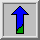# Bitshift Operators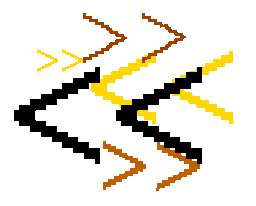Common Names: Bitshifting

## Brief Description

The bitshift operator works on images represented in byte or integer pixel format, where each pixel value is stored as a binary number with a fixed amount of bits. Bitshifting shifts the binary representation of each pixel to the left or to the right by a pre-defined number of positions. Shifting a binary number by one bit is equivalent to multiplying (when shifting to the left) or dividing (when shifting to the right) the number by 2.## How It Works

The operation is performed straightforwardly in a single pass. If the binary representation of a number is shifted in one direction, we obtain an empty position on the opposite side. There are generally three possibilities of how to fill in this empty position: we can pad the empty bits with a 0 or a 1 or we can wrap around the bits which are shifted out of the binary representation of the number on the other side. The last possibility is equivalent to rotating the binary number.

The choice of technique used depends on the implementation of the operator and on the application. In most cases, bitshifting is used to implement a fast multiplication or division. In order to obtain the right results for this application, we have to pad the empty bits with a 0. Only in the case of dividing a negative number by a power of 2, do we need to fill the left bits with a 1, because a negative number is represented as thetwo's-complement of the positive number, i.e. the sign bit is a 1. The result of applying bitshifting in this way is illustrated in the following formula: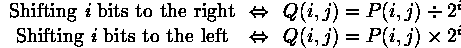An example is shown in Figure 1.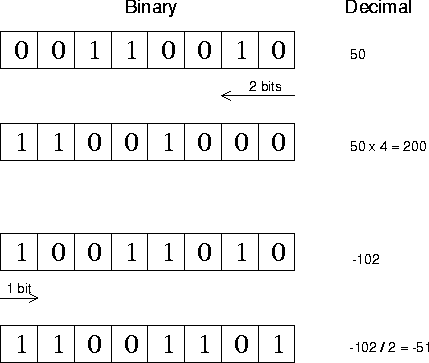Figure 1 Examples for using bitshifting for multiplication and division. Note that the bottom example uses a signed-byte convention where a byte represents a number between -128 and +127

If bitshifting is used for multiplication, it might happen that the result exceeds the maximum possible pixel value. This is the case when a 1 is shifted out of the binary representation of the pixel value. This information is lost and the effect is that the value is wrapped around from zero.## Guidelines for Use

The main application for the bitshift operator is to divide or multiply an image by a power of 2. The advantage over the normal pixel division and pixel multiplication operators is that bitshifting is computationally less expensive.

For example, if we want to add two images we can use bitshifting to make sure that the result will not exceed the maximum pixel value. We illustrate this example using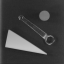and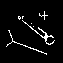where the latter is the skeleton gained from the thresholded version of the former. To better visualize the result of the skeletonization we might want to overlay these two images. However, if we add them straightforwardly we obtain pixel values greater than the maximum value. First shifting both images to the right by one bit yields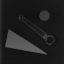and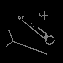which then can be added without causing any overflow problems. The result can be seen in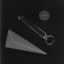Here, we can see that shifting a pixel to the right does, as a normal pixel division, decrease the contrast in the image.

On the other hand, shifting the binary representation of a pixel to the left increases the image contrast, like the pixel multiplication. For example,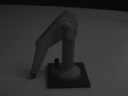is an image taken under poor lighting conditions. Shifting each pixel in the image to the left by one bit, which is identical to multiplying it with 2, yields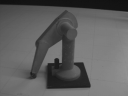Although the operator worked well in this example, we have to be aware that the result of the multiplication might exceed the maximum pixel value. Then, the effect for the pixel value is that it is wrapped around from 0. For example, if we shift each pixel in the above image by two bits, at some pixels a 1 is shifted out of the binary representation of the image, resulting in a loss of information. This can be seen in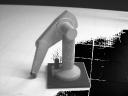In general, we should make sure that the values in the input image are sufficiently small or we have to be careful when we interpret the resulting image. Alternatively, we can change the pixel value format prior to applying the bitshift operator, e.g. change from byte format to integer format.

Although multiplication and division are the main applications for bitshifting it might also be used for other, often very specialized, purposes. For example, we can store two 4-bit images in a byte array if we shift one of the two images by 4 bits and mask out the unused bits. Using the logical OR operator we can combine the two images into one without losing any information. Sometimes it might also be useful to rotate the binary representation of each bit, apply some other operator to the image and finally rotate the pixels back to the initial order.

## Interactive Experimentation

You can interactively experiment with this operator by clicking here.

## Exercises

1. Use pixel addition to overlayand its edge image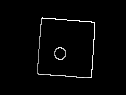Apply the bitshift operator to the original image in order to increase its contrast. Convert the image into an integer format prior to the shifting to preserve all image information. Compare the result of the addition with the one you get without the bitshifting.

2. What is the result of dividing -7 (binary:1001) by 2 using bitshifting. What is the result of dividing +7 (binary:0111) by 2?

## References

E. Davies Machine Vision: Theory, Algorithms and Practicalities, Academic Press, 1990, Chap. 2.

R. Gonzalez and R. Woods Digital Image Processing, Addison-Wesley Publishing Company, 1992, pp 50 - 51.

## Local Information